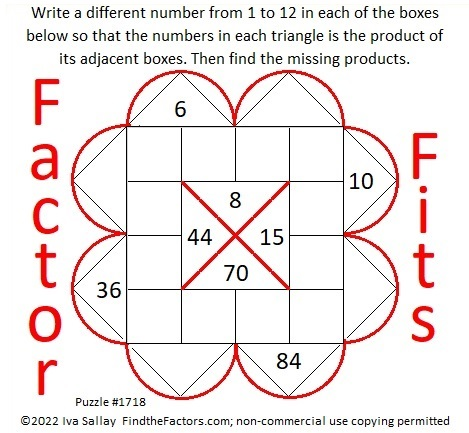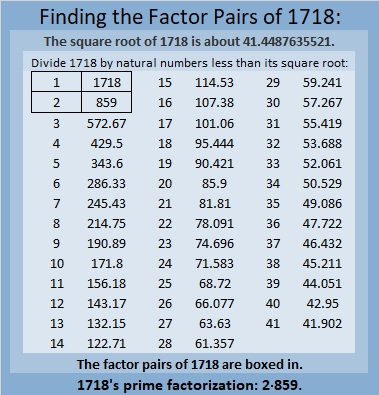# 1718 Factor Fits Valentine

Contents

### Today’s Puzzle:

Happy Valentine’s Day! I hope you enjoy my Valentine to YOU!### Other Mathematical Valentines:

Colleen Young of Mathematics, Learning and Technology has a nice collection of mathematical hearts For Valentine’s Day.

### Factors of 1718:

• 1718 is a composite number.
• Prime factorization: 1718 = 2 × 859.
• 1718 has no exponents greater than 1 in its prime factorization, so √1718 cannot be simplified.
• The exponents in the prime factorization are 1 and 1. Adding one to each exponent and multiplying we get (1 + 1)(1 + 1) = 2 × 2 = 4. Therefore 1718 has exactly 4 factors.
• The factors of 1718 are outlined with their factor pair partners in the graphic below.### More About the Number 1718:

1718 is the sum of four consecutive numbers:
428 + 429 + 430 + 431 = 1718.

This site uses Akismet to reduce spam. Learn how your comment data is processed.